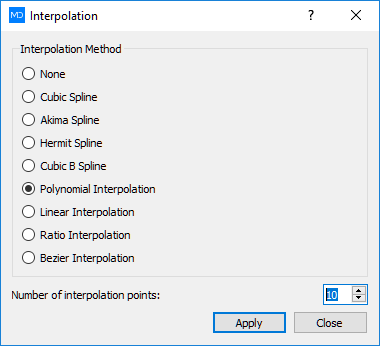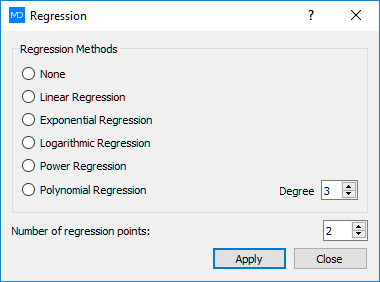# Curve Fitting

MatDeck has curve fitting facilities which from a series of data points you can apply various interpolations and regressions.

## Interpolations

MatDeck’s interpolation curve fitting gives you an exact fit to the available series of data points.

Available interpolations are:

• Linear interpolation
• Polynomial interpolation
• Ration interpolation
• Cubic spline
• Akima spline
• Hermite spline
• Cubic B spline
• Bezier interpolation## Regressions

MatDeck’s regression curve fitting generates a curve based on statistical processes for estimating the relationships among a series of data points.

Available regressions:

• Linear
• Exponential
• Logarithmic
• Power regression
• Polynomial regressionMatDeck gives you two different technologies for curve fittings. The first one is a classical approach where the user is using a standard set of curve fitting functions and commands showed in MatDeck document Curve fitting. The second technology is a curve fitting tool box shown in MatDeck’s Curve fitting toolkit document. With MatDeck parallel processing, Excel, Database interface and other MatDeck features the user gets an extremely advanced but simple solution for curve and function fitting. Generated curve fitted data and functions are available as curve plotting graph, curve function or an array of curve data.